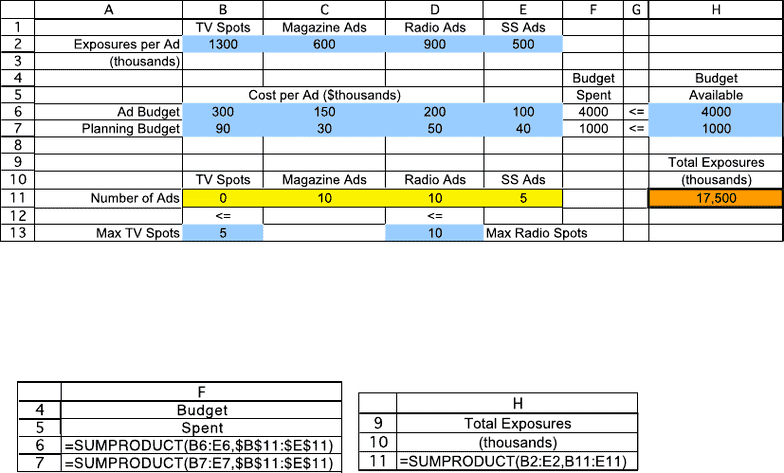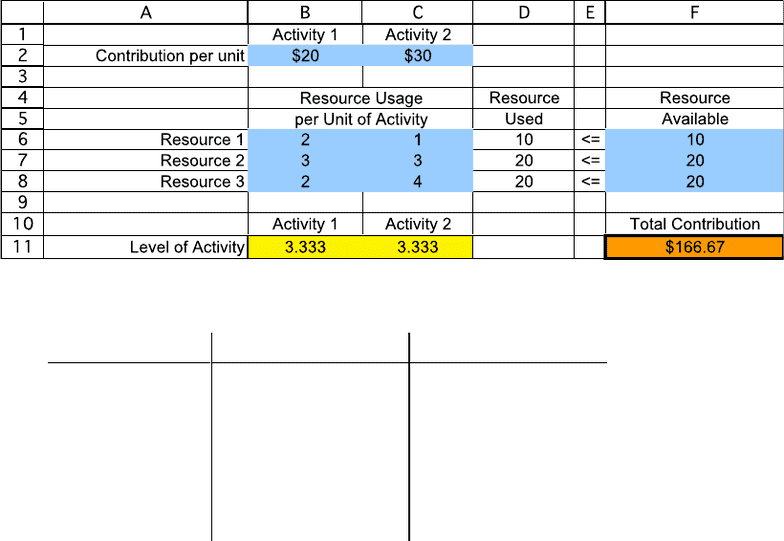Textbook Notes (280,000)
CA (170,000)
UOttawa (6,000)
Chapter 3

# ADM 2302 Chapter Notes - Chapter 3: Lincoln Near-Earth Asteroid Research, Thinkpad X Series

Department
Course Code
Professor
Rim Jaber
Chapter
3

This preview shows page 1. to view the full 5 pages of the document.Week 3 Extra Problems Solution
CHAPTER 3
LINEAR PROGRAMMING: FORMULATION AND
APPLICATIONS
Problems
3.1
a)
Data cells: B2:E2, B6:E7, H6:H7, B13, and D13
Changing cells: B11:E11
Objective cell: H11
b) This is a linear programming model because the decisions are represented by changing cells that
can have any value that satisfy the constraints. Each constraint has an output cell on the left, a
mathematical sign in the middle, and a data cell on the right. The overall level of performance is
represented by the objective cell and the objective is to maximize that cell. Also, the Excel
equation for each output cell is expressed as a SUMPRODUCT function where each term in the
sum is the product of a data cell and a changing cell.
c) Let T = number of commercials on TV
R = number of commercials on radio

Unlock to view full version

Only page 1 are available for preview. Some parts have been intentionally blurred.ADM2302 Solution for Extra Problems Week 3
2
d) Maximize Exposures (thousands) = 1300T + 600M + 900R + 500S
subject to 300T + 150M + 200R + 100S 4,000 (\$thousands)
90T + 30M + 50R + 40S ≤ 1,000 (\$thousands)
T ≤ 5 spots
R ≤ 10 spots
and T ≥ 0, M ≥ 0, R ≥ 0, S ≥ 0.
3.3
a) and c)
b)
(x1, x2)
Feasible?
Total Contribution
(2,2)
Yes
\$100
(3,3)
Yes
\$150
(2,4)
Yes
\$160
Best
(4,2)
Yes
\$140
(3,4)
No
(4,3)
No
d) Let x1 = level of activity 1
x2 = level of activity 2
Maximize Contribution = \$20x1 + \$30x2
subject to 2x1 + x2 ≤ 10
3x1 + 3x2 ≤ 20
2x1 + 4x2 20
and x1 ≥ 0, x2 ≥ 0.